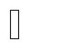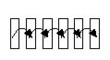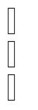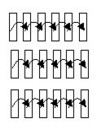# Solving scenario / computation step: about

## Scenario: definition

A solving scenario is a Flux entity, which defines a control of the solving process (see example below).

## Examples

• In a Flux transient application the solving scenario sets up the time control, i.e. the values of time steps.
• Within a parametric analysis, the solving scenario sets up the control of parameters, i.e. the values of parameters for various cases (computation steps).

## Types of scenarios

Various types of scenarios in function of the study type (with / without parameters) and of the application type (transient / non-transient) are possible; they are presented in the table below.

Analysis without parameters
Application System … Scenario
Non transient Static / Harmonic / Quasi static

linear / non linear

Mono step

(one step)Transient Transient

linear / non linear

+

transient

Transient multi step

(More steps «connected to each other»)Analysis with parameters
Application System … Scenario
Non transient Static / Harmonic / Quasi static

linear / non linear

Parametric multi step

(Several independent steps)Transient Transient

linear / non linear

+

transient

Transient and parametric multi step

(Several steps «connected to each other» or independent)Note: There is always a solving scenario, even for the case when there is only one computation step.

## Complements

There can be more than one scenario in the same Flux project.

## Scenario states

States of a solving scenario can be as follows:

• unprocessed (not processed yet)
• partly processed (some steps are processed and some not)
• fully processed (all the steps are processed)

## Computation step states

States of a computation step are presented in the table below.

State Example Post-processing
unprocessed solving process uncompleted or completed with user's stop at the end of the step / adding a step to the scenario no
processed incorrectly solved

problem of convergence

user's stop at the end of an iteration

yes
unsolved

error in modeling

no
correctly solved   yes